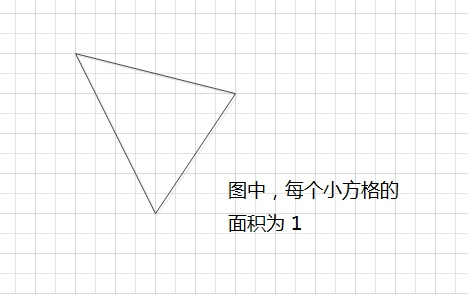# 第六届蓝桥杯大赛个人赛省赛（软件类） Java 大学B组第二题：

1^3 = 1
8^3  = 512    5+1+2=8
17^3 = 4913   4+9+1+3=17
...

package two;

public class Main {

public static long sum(long a){
long sum=0;
while(a/10>0){
sum+=a%10;
a/=10;
}
sum+=a;
return sum;
}

public static void main(String[] args) {
int count=0;
for(int i=1;i<10000;i++){
if(i==sum((int)Math.pow(i, 3))){
count++;
System.out.println(i);
}
}
System.out.println("共"+count);
}
}


输出：
1
8
17
18
26
27

祥 瑞 生 辉
+  三 羊 献 瑞
-------------------
三 羊 生 瑞 气

(如果有对齐问题，可以参看【图1.jpg】)

9587
+ 1085
10652

public static int f(int n, int m)
{
n = n % m;
Vector v = new Vector();

for(;;)
{
n *= 10;
n = n % m;
if(n==0) return 0;
if(v.indexOf(n)>=0)  _________________________________ ;  //填空
}
}

1,2,3...9 这九个数字组成一个分数，其值恰好为1/3，如何组法？

public class A
{
public static void test(int[] x)
{
int a = x*1000 + x*100 + x*10 + x;
int b = x*10000 + x*1000 + x*100 + x*10 + x;
if(a*3==b) System.out.println(a + " " + b);
}

public static void f(int[] x, int k)
{
if(k>=x.length){
test(x);
return;
}

for(int i=k; i<x.length; i++){
{int t=x[k]; x[k]=x[i]; x[i]=t;}
f(x,k+1);
_______________________________________       // 填空
}
}

public static void main(String[] args)
{
int[] x = {1,2,3,4,5,6,7,8,9};
f(x,0);
}
}

1+2+3+...+10*11+12+...+27*28+29+...+49 = 2015

package six;
/**
* 2015年4月11日14:51:20
* @author 图乐
*
*/
public class Main {
public static void fun(int n,int sum,int count,char last,StringBuffer sb){
if(n<50&&sum>=0){
sb.append(n);

if(count>0&&last!='*'){
fun(n+1,sum-n*(n+1),count-1,'*',new StringBuffer(sb).append("*"));
}
if(last=='*')
fun(n+1,sum,count,'+',new StringBuffer(sb).append("+"));
else
fun(n+1,sum-n,count,'+',new StringBuffer(sb).append("+"));
}
if(n==50&&sum==0&&count==0){
System.out.println("ok:"+sb.toString());
}
}

public static void main(String[] args) {
fun(1, 2015, 2, ' ',new StringBuffer());
}

}

输出：
ok:1+2+3+4+5+6+7+8+9+10*11+12+13+14+15+16+17+18+19+20+21+22+23+24+25+26+27*28+29+30+31+32+33+34+35+36+37+38+39+40+41+42+43+44+45+46+47+48+49+
ok:1+2+3+4+5+6+7+8+9+10+11+12+13+14+15+16*17+18+19+20+21+22+23+24*25+26+27+28+29+30+31+32+33+34+35+36+37+38+39+40+41+42+43+44+45+46+47+48+49+

100

149

101

151

CPU消耗  < 1000ms

10000

14999

3559

package eight;
import java.util.Scanner;

/**
*
* 2015年4月11日14:56:25

* @author 图乐
*
*/
public class Main {
//int [][] save = new int;
public static void main(String[] args) {
Scanner in = new Scanner(System.in);
long s = System.currentTimeMillis();
System.out.println(f(in.nextInt()));
long e = System.currentTimeMillis();
System.out.println((e-s));
}
public static int  f(int n) {
int count=0;
int yushu=0;
while(n>0){
count+=n;
int temp=n;
n=(n+yushu)/3;
yushu=(temp+yushu)%3;

}
return count;
}
}<span style="color:#ff0000;">
</span>

「输入格式」

n表示骰子数目

「输出格式」

「样例输入」
2 1
1 2

「样例输出」
544

「数据范围」

CPU消耗  < 2000ms

「输入格式」

「输出格式」

「样例输入」
5
1 -2 -3 4 5
4 2
3 1
1 2
2 5

「样例输出」
8

「数据范围」

CPU消耗  < 3000ms

02-26178

#### 第十届蓝桥杯大赛软件类省赛 Java 大学 B组 题解

06-041万+

#### 第十届蓝桥杯JavaB组省赛真题04-073万+

#### 电网101、104规约解析（Java）

03-283286

#### 2019年蓝桥杯省赛Java-B组

05-271万+

#### 2018第九届蓝桥杯省赛，国赛参赛经验心得分享（JavaB组）

02-21134

#### 蓝桥杯2019年省赛JAVA大学B组

01-276607

#### jav开放地址法和链地址法解决hash冲突

04-159964

#### 2019第十届蓝桥杯JavaB组省赛真题详解

03-301323

#### 第十届(2019)蓝桥杯JavaB组习题讲解©️2020 CSDN 皮肤主题: 大白 设计师: CSDN官方博客点击重新获取扫码支付1.余额是钱包充值的虚拟货币，按照1:1的比例进行支付金额的抵扣。
2.余额无法直接购买下载，可以购买VIP、C币套餐、付费专栏及课程。余额充值